Get instant live expert help with Excel or Google Sheets“My Excelchat expert helped me in less than 20 minutes, saving me what would have been 5 hours of work!”

#### Post your problem and you’ll get Expert help in seconds.

Your message must be at least 40 characters
Our professional Expert are available now. Your privacy is guaranteed.

# Excel ISODD Function

We can use the Excel ISODD function to test whether an imputed numerical value is an odd number. This article will walk through the simple steps.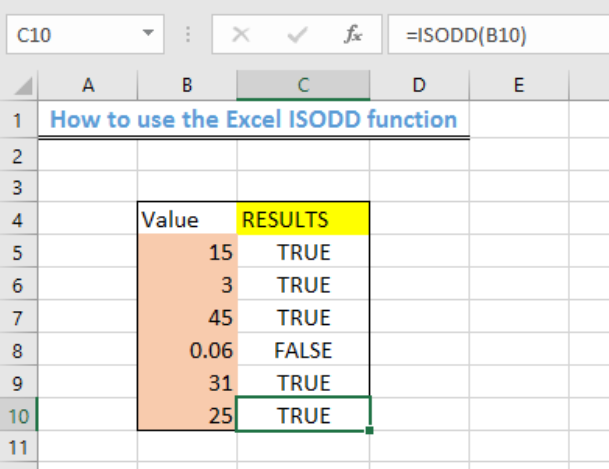Figure 1 – How to Use the Excel ISODD function

## General Formula

`=ISODD(value)`

• “Value” is the numerical value we want to test if it is ODD

## Formula

`=ISODD(B5)`

## Setting up the Data

We will structure our table in the pattern as shown in figure 2. We want to check if each number under the “value” column is an odd number.

• We will input the values in the range B5:B10
• The RESULTS will be displayed in Column C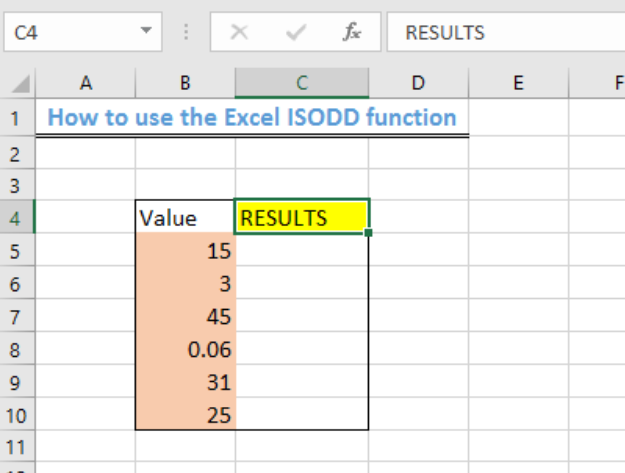Figure 2 – Setting up the data

## Using the Excel ISODD function

• We will click on Cell C5
• We will insert the formula below into C5

`=ISODD(B5)`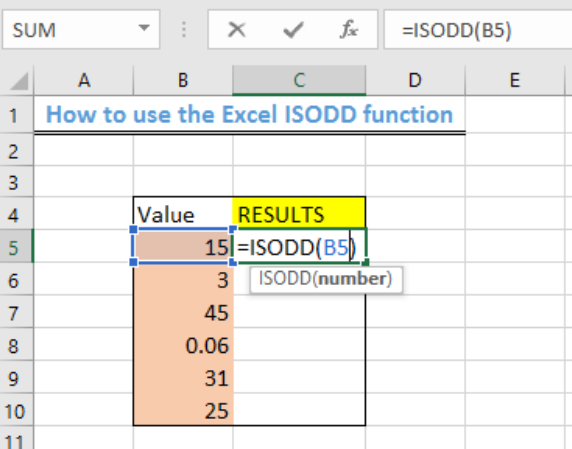Figure 3 – Applying the Excel ISODD function

• We will press the enter key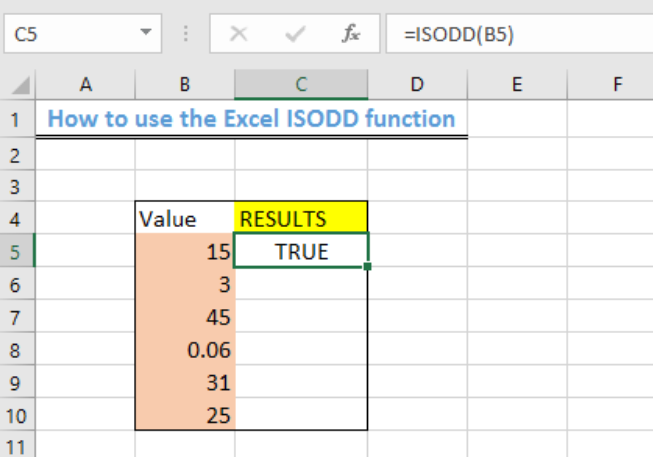Figure 4- How to use the Excel ISODD function

• We will use the fill handle to copy the formula into the remaining cells to get the result.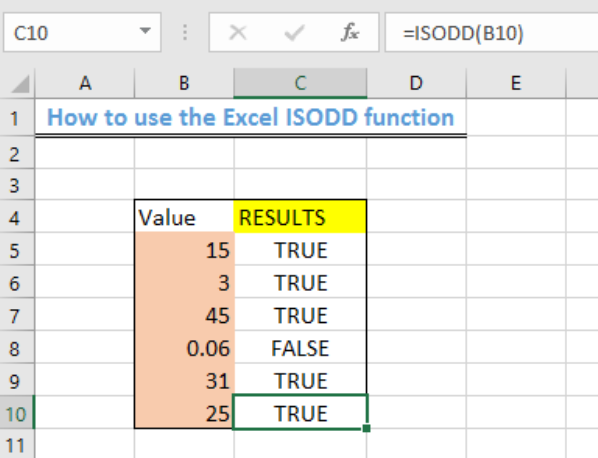Figure 5 – Final Result of applying the Excel ISODD function

## Explanation

The ISODD function checks if the specified value or cell reference has an ODD number and returns the result with a TRUE or FALSE. If there is no numeric value in the cell, then the ISODD function will return the #VALUE error.

## Instant Connection to an Expert through our Excelchat Service

Most of the time, the problem you will need to solve will be more complex than a simple application of a formula or function. If you want to save hours of research and frustration, try our live Excelchat service! Our Excel Experts are available 24/7 to answer any Excel question you may have. We guarantee a connection within 30 seconds and a customized solution within 20 minutes.

Solution examplesI need to count number of dates from a timestamped date in column from the completed date column to show the number of days. I have not successfully been able to do this. I am a novice Excel user. Can you help?
Solved by O. B. in 15 minsUse the Vlookup Function to complete the "employee" column of table 2. Use "job Id" from table 2 as your lookup_value(s) and table 1 as your reference.
Solved by C. H. in 16 minsIf a cell in another sheet is populated I need a vlookup done. If the cell is not populated I need the cell to return blank.
Solved by T. D. in 60 minsI am trying to make a chart that turns a week range red if nothing is entered in the range. If something is entered then I would like it to turn green. Please Help
Solved by E. U. in 43 minsI need a check box to show/hide an answer of an if function
Solved by Z. U. in 23 mins